Monday, December 31, 2018

Andrew Ridgeley crashes at Brands

It's the 1986 Cellnet Superprix, the rain is relentless, and it's dark, very dark. Too dark, in fact, for those amateur photographers foolish enough to have arrived with nothing other than 100 ASA film...

The field is strong, containing future luminaries such as Martin Donnelly, Perry McCarthy, Damon Hill, Gary Brabham, Andy Wallace, David Leslie and Julian Bailey. Also entered is Andrew Ridgeley, George Michael's partner in contemporary pop-duo Wham!Failing to compensate for the reduction in the effective power-spectrum of the road-surface, Ridgeley Go-Goes into the gravel at Druids...His momentum is somewhat checked by the Cellnet cars of Ross Cheever and David Hunt, which have already found the tyre-wall.Nothing for it but to head back to the Kentagon for a warming cup of tea.

Friday, December 28, 2018

Brands Hatch F3000 1987

Second installment of the McCabism photographic archive. The place once again is Brands Hatch, this time for a round of the European F3000 championship. By 1987 the Grand Prix had moved to the numbing wind-swept expanses of Silverstone, so this was very much Brands' biggest single-seater race of the year.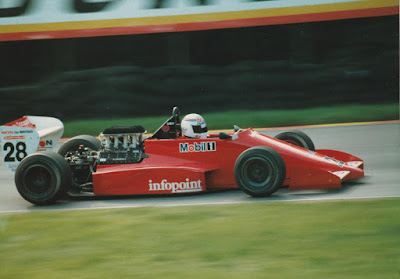F3000 cars of the era tended to be sans engine cover. This is the Madgwick Motorsport Lola of Andy Wallace.The absent engine cover facilitated tall intake trumpets, which maximise torque at lower revs, F3000 engines being restricted to 9,000rpm. (When the intake valve opens, it creates a rarefaction wave, which propagates to the top of the open-ended trumpet, and reflects as a compression wave. The lower the revs, the longer the necessary trumpet length so that the compression wave returns just as the intake valve is closing, pushing more mass into the combustion chamber). This is the Pavesi Racing Ralt of Pierluigi Martini.This is a motor racing circuit. It possesses gradient and contour, exists in a natural setting, and is distinguishable from a go-kart track.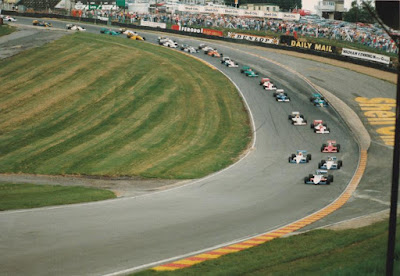First corner of the race, and Julian Bailey has immediately overtaken the front-row sitters, Gugelmin and Moreno in their Ralts.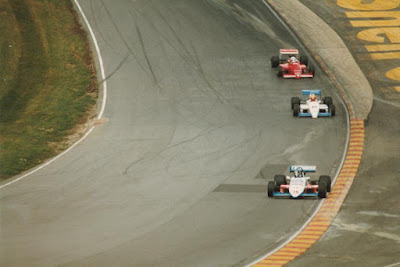Andy Wallace moves past Moreno into 3rd, but Bailey looks comfortable in the lead.Stefano Modena ahead of Yannick Dalmas, the latter displaying a black tyre-mark on his nose-cone as evidence of prior contact with Modena. Dalmas had tapped Modena into a spin at Druids, but courteously waited for Modena to resume ahead of him.Michel Trolle has a territorial dispute at Druids with A Yorkshireman. This inevitably results in a large accident, Trolle's car flipping upside down onto the top of the tyre-wall.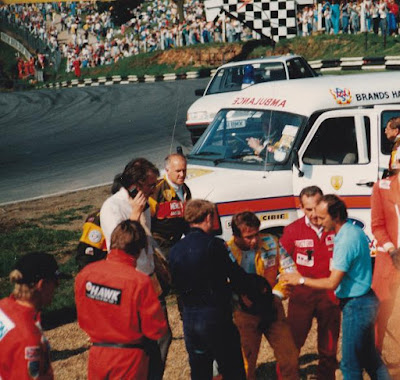A slightly shocked Trolle stumbles to his feet after being hauled from the upturned car.The race is red-flagged and Bailey awarded victory.

Sunday, December 23, 2018

Brands Hatch Tyre Test 1986

Time to dip into the McCabism personal photographic archive. The occasion here is the 1986 pre-Grand Prix Tyre Test at Brands Hatch.

Proper drivers, proper cars, and a proper circuit.Nigel Mansell zipping down the pit-straight in a car weighing less than 700kg.Stefan Johansson's Ferrari, in the days before people from Northern Europe and South Africa introduced the Scuderia to the exciting world of stable balanced downforce.Nelson Piquet, plotting and scheming as he brakes for the hairpin at Druids.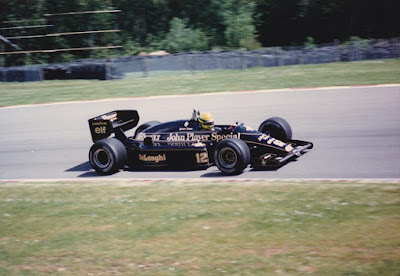Ayrton Senna negotiating the curved flow at Hawthorns.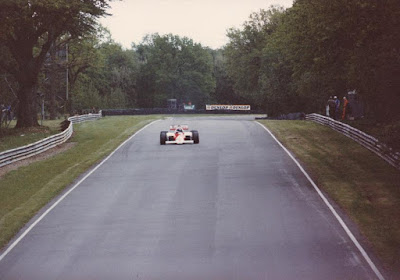Poking my camera over the bridge parapet to get a shot of Keke Rosberg accelerating down the hill to Clearways.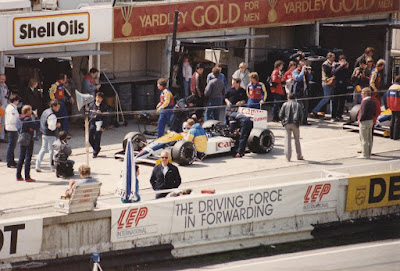Nigel Mansell in the pits, complaining of an excessive surface/bulk tyre temperature delta, and some inconsistent behaviour from the Y250 vortex.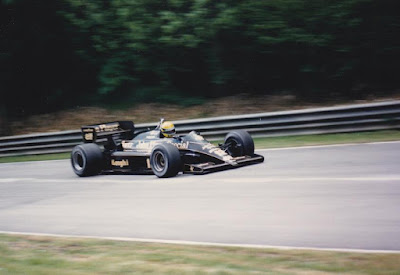Ayrton Senna cresting the rise after the sylvan delights of Dingle Dell, and preparing to turn into Dingle Dell corner.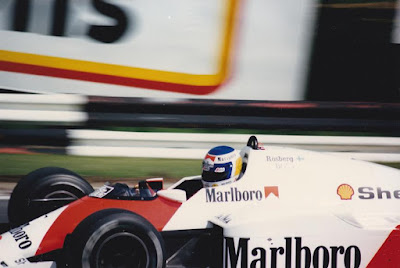Keke Rosberg in the McLaren, somehow living to tell the tale without a halo for protection.

Thursday, July 19, 2018

Understanding tyre compound deltas

Pirelli revealed at the beginning of the 2018 F1 season that it was using new software to help it choose the three tyre compounds available at each race. Pirelli's Racing Manager Mario Isola commented:

"It's important that we collect the delta lap times between compounds to decide the selection. If we confirm the numbers that we have seen in Abu Dhabi [testing in November] - between soft and supersoft we had 0.6s, and supersoft to ultrasoft was 0.4s - depending on that, we can fine tune the selection and try to choose the best combination."

Getting the tyre compound deltas correct is indeed a crucial part of F1 race strategy, so let's review some of the fundamental facts about these numbers. The first point to note is that tyres are a performance multiplier, rather than a performance additive

To understand this in the simplest possible terms, consider the following equation:
$$F_y = \mu F_z$$ This states that the lateral force $F_y$ generated by a tyre is a product of the coefficient of friction $\mu$, and the vertical load $F_z$. All other things being equal, the greater the lateral force generated by a car in the corners, the faster the laptime. (Note, however, that in many circumstances one would wish to work with lateral acceleration rather than lateral force, given the influence of car-mass on lateral acceleration).

Now, suppose we have a base compound. Let's call it the Prime, and let's denote its coefficient of friction as $\mu_P$. Let's consider a fixed car running the Prime tyre with: (i) a light fuel-load, and (ii) a heavy fuel-load.

Let's really simplify things by supposing that the performance of the car, and its laptime, can be reduced to a single vertical load due to downforce alone, and a single lateral force number. When the car is running a heavy fuel load, it will generate a downforce $F_z$, but when it's running a light fuel load it will be cornering faster, so the vertical load due to downforce will be greater, $F_z + \delta F_z$. (Recall that the contribution of greater fuel weight to vertical load results in a net loss of lateral acceleration due to weight transfer). The lateral forces will be as follows:

$\mu_P F_z$

$\mu_P (F_z + \delta F_z) = \mu_P F_z + \mu_P\delta F_z$

Now, let's suppose that there is a softer tyre compound available. Call it the Option. Its coefficient of friction $\mu_O$ will be greater than that of the Prime, $\mu_O = \mu_P + \delta \mu$.

Consider the performance of the same car on the softer compound, again running a light fuel-load and a heavy fuel-load:

$\mu_O F_z = ( \mu_P +\delta \mu ) F_z$

$\mu_O (F_z + \delta F_z) = ( \mu_P +\delta \mu )(F_z + \delta F_z)$

So far, so good. Now let's consider the performance deltas between the Option and the Prime, once again using lateral force as our proxy for laptime.

High-fuel Option-Prime delta

$( \mu_P +\delta \mu ) F_z-\mu_P F_z = \delta \mu F_z$

Low-fuel Option-Prime delta

$( \mu_P +\delta \mu )(F_z + \delta F_z)-\mu_P (F_z + \delta F_z)=\delta \mu (F_z + \delta F_z)$

Notice that sneaky extra term, $\delta \mu \delta F_z$, in the expression for the low-fuel compound delta? As a consequence of that extra term, the Option-Prime delta is greater on a low fuel load than a heavy fuel-load. As promised, tyre-grip is a performance multiplier.

If you scrutinise the compound deltas in each FP2 session, you'll see that the low-fuel compound deltas from the beginning of the session are indeed greater than those from the high-fuel running later in the session.

Given that the compound deltas input into race-strategy software are generally high-fuel deltas, one could make quite a mistake by using those low-fuel deltas. In fact, parties using low-fuel deltas might be surprised to see more 1-stop races than they were expecting.

There is another important consequence of the fact that tyres are performance multipliers: the pace gap between faster cars and slower cars increases when softer tyres are supplied. The faster cars have more downforce, and therefore more vertical load $F_z$ than the slower cars, at any equivalent fuel-weight. The delta in vertical load is multiplied by the delta in the coefficient of friction, and all things being equal, the faster cars duly benefit from that extra $\delta \mu \delta F_z$.

Of course, that qualification about 'all things being equal', hides some complex issues. For example, softer tyres have a lower 'cornering stiffness', (i.e., the gradient of lateral force against slip-angle). A softer tyre therefore generates peak grip at a higher slip-angle than a harder tyre. If the aerodynamics of a car are particularly susceptible to the steering angle of the front wheels, then such a car might struggle to gain proportionately from the greater grip theoretically afforded by a softer tyre. Such a car would also appear to gain, relative to its opposition, towards the end of a stint, when the tyres are worn and their cornering stiffness increases.

Notwithstanding such qualifications, the following problem presents itself: the softer the tyres supplied to the teams in an attempt to enhance the level of strategic variety, the greater the pace-gaps become, and the less effect that strategic variety has...

Tuesday, May 15, 2018

Front-wing in yaw

Armchair aerodynamicists might be interested in a 2015 paper, 'Aerodynamic characteristics of a wing-and-flap in ground effect and yaw'.

The quartet of authors from Cranfield University analyse a simple raised-nose and front-wing assembly, consisting of a main-plane and a pair of flaps, equipped with rectangular endplates. On each side of the wing, three vortices are created: an upper endplate vortex, a lower endplate vortex, and a vortex at the inboard edge of the flap. (The latter is essentially a weaker version of the Y250 which plays such an important role in contemporary F1 aerodynamics).

The authors assess their front-wing in yaw, using both CFD and the wind-tunnel, and make the following observations:

1) In yaw, vortices generated by a lateral movement of air in the same direction as the free-stream, increase in strength, whereas those which form due to air moving in the opposite direction are weakened.

2) The leeward side of the wing generates more downforce than the windward side. This is due to an increase in pressure on the leeward pressure surface and a decrease in suction on the windward suction surface. The stagnation pressure is increased on the inner side of the leeward endplate, and the windward endplate partially blocks the flow from entering the region below the wing.

3) A region of flow separation occurs on the windward flap suction surface.

4) Trailing edge separation occurs in the central region of the wing. This is explained by the following: (i) The aluminium wing surface was milled in the longitudinal direction, hence there is increased surface roughness, due to the material grain, for air flowing spanwise across the surface; (ii) There is a reduction in the mass flow-rate underneath the wing; (iii) The effective chord-length has increased in yaw.

5) The vortices follow the free-stream direction. Hence, for example, the windward flap-edge vortex is drawn further towards the centreline when the wing is in yaw.

One comment of my own concerns the following statement:

"The yaw rate for a racing car can be high, up to 50°/sec, but is only significant aerodynamically during quick change of direction events, such as initial turn-in to the corner. The yaw angle, however, is felt throughout the corner and is usually in the vicinity of 3-5°. Although the yaw angle changes throughout the corner the yaw rate is not sufficiently high, other than for the initial turn-in event, to warrant any more than quasi-static analysis."

This is true, but it's vital to point out that the stability of a car in the dynamic corner-entry condition determines how much speed a driver can take into a corner. If the car is unstable at the point of corner-entry, the downforce available in a quasi-static state of yaw will be not consistently accessible.

Aerodynamicists have an understandable tendency to weight conditions by their 'residency time'. i.e., the fraction of the grip-limited portion of a lap occupied by that condition. The fact that the high yaw-rate corner-entry condition lasts for only a fraction of a second is deceptive. Minimum corner speed depends not only on the downforce available in a quasi-static state of yaw, but whether the driver can control the transition from the straight-ahead condition to the quasi-static state of yaw.

Sunday, April 29, 2018

Local cosmography and the Wiener filter

The local cosmic neighbourhood has recently been mapped in spectacular fashion by the Cosmicflows research programme, yielding papers in Nature, and an article in Scientific American. The images generated are stunning.Perspective view of the X-Y equatorial plane of our cosmic neighbourhood in supergalactic coordinates. The density of matter is represented by colour contours, deep blue regions indicating voids, red indicating zones of high density. The velocity streams are represented in white, with individual galaxies as spheres.
There's also an interesting mathematical back-story here because the work has been underpinned by techniques developed over 20 years ago by Yehuda Hoffman and colleagues. Drawing upon the exposition provided by Hoffman, let's take a look at these methods, beginning with Gaussian fields.

Gaussian fields

A random field is a field in which the value at each point is sampled from a univariate probability distribution, and the values at multiple points are sampled from a multivariate distribution. Typically, the sampled values at different points are spatially correlated, with a degree of correlation that declines with distance.

A Gaussian field is a type of random field in which the distribution at each point is Gaussian, and relationship between the values at different points is given by a multivariate Gaussian distribution. The properties of a Gaussian field are specified by its covariance matrix. Assuming a Gaussian field $\mathbf{s}$ of zero mean, this is denoted as:
$$\mathbf{S} = \langle \mathbf{s} \mathbf{s}^T\rangle$$ Treating $\mathbf{s}$ as a vector of random variables, this expression is understood as the 'outer-product' of the column vector $\mathbf{s}$ with its transpose row vector $\mathbf{s}^T$:
$$\mathbf{S} = {\langle s_i s_j \rangle}$$Convolution

Given the Gaussian field $\mathbf{s}$, the generation of the measured data-set $\mathbf{d}$  is represented as follows:
$$\mathbf{d} = \mathbf{Rs} + \mathbf{\epsilon}$$ $\mathbf{R}$ represents the characteristics of the measuring instrument, and $\epsilon$ represents measurement noise. The covariance matrix of the noise is denoted as follows:
$$\mathbf{N} = \langle \mathbf{\epsilon} \mathbf{\epsilon}^T\rangle$$ The transformation $\mathbf{R}$ is typically a convolution. In astronomical measurements of the sky it is often referred to as the Point Spread Function. It specifies how the energy detected to belong to a particular pixel of the sky is actually a weighted sum of the energy belonging to a range of adjacent pixels. Similarly, in spectroscopy it specifies how the energy detected at each particular wavelength is actually a weighted sum of the energy belonging to adjacent wavelengths, thereby smearing out the energy spectrum.

Given the true spectrum $f(\lambda)$, and the convolution kernel $g(\lambda - \lambda_1)$, the measured spectrum $I(\lambda)$ is defined by the convolution:
$$I(\lambda) = f * g = \int f(\lambda_1) g(\lambda - \lambda_1) d\lambda_1$$
Wiener filter

Stochastic filtering provides a collection of techniques for constructing estimates of the true values of a quantity or field from sparse and noisy measurement data. The Wiener filter is one such technique. It provides a transformation $\mathbf{F}$ that maps a measured dataset $\mathbf{d}$ into an estimate of the true field:
$$\mathbf{s}^{WF} = \mathbf{F} \mathbf{d}$$ The discrepancy between the true field and the estimated field is called the residual:
$$\mathbf{r} = \mathbf{s} - \mathbf{s}^{WF}$$ The residual possesses its own covariance matrix $\langle \mathbf{r}\mathbf{r}^T \rangle$. The Wiener filter is defined so that it minimizes the covariance of the residual.

The Wiener filter possesses another property which makes it the natural choice of filter for cosmography: Given the prior distribution of the true field $\mathbf{s}$; given the relationship between the true field and the measured values; and given the measured values, a posterior Bayesian distribution $p(\mathbf{s}|\mathbf{d})$ can be obtained over the true field. In the case where the true field is a Gaussian field, and the noise is also Gaussian, the Wiener filter picks out the mean value of the posterior distribution.

The Wiener filter is given by the expression:
$$\mathbf{F} = \mathbf{SR}^T (\mathbf{RSR}^T + \mathbf{N})^{-1}$$
Constrained realizations

The method of constrained realizations goes back to a paper by Yehuda Hoffman and Erez Ribak in 1991 ('Constrained realizations of Gaussian fields - A simple algorithm', Ap. J. Lett., vol 380, pL5-L8). It's based upon a simple but crucial point: the application of the Wiener filter to a measured dataset will produce an estimated field which includes the covariance of the residual: $$\mathbf{s}^{WF} = \mathbf{s} - \mathbf{r}$$
Hence, the estimated field will be smoother than the true field. The idea proposed by Hoffman and Ribak is simple: to generate a realistic realization of the true field, you need to add a sampled realization from the residual field.

Their method works as follows:

(i) Generate a random realization $\tilde{\mathbf{s}}$ of the true field. (The tilde here indicates a realization).

(ii) Generate a realization of the measurement noise, and apply the measurement transformation to $\tilde{\mathbf{s}}$ to yield a random dataset realization: $$\mathbf{\tilde{d}} = \mathbf{R\tilde{s}} + \mathbf{\tilde{\epsilon}}$$
(iii) Apply the Wiener filter to $\mathbf{\tilde{d}}$ to create an estimate of the true field realization: $\mathbf{F} \mathbf{\tilde{d}}$.

(iv) Generate a realization of the residual:
$$\mathbf{\tilde{r}} = \tilde{\mathbf{s}} - \mathbf{F} \mathbf{\tilde{d}}$$
(v) Add the realization of the residual to the estimated field which has been obtained by applying the Wiener filter to the actual measured dataset:
\eqalign{\mathbf{s}^{CR} &= \mathbf{\tilde{r}} + \mathbf{Fd} \cr &=\tilde{\mathbf{s}}+ \mathbf{F} (\mathbf{d-\tilde{d}})}
Reconstruction of continuous fields from finite data

Here the Gaussian field $\mathbf{s}$ is assumed to be a function $f(\mathbf{r})$, so that the covariance matrix becomes the auto-correlation function $\xi$:

$$\mathbf{S} = \langle f(\mathbf{r}_i) f(\mathbf{r}_j)\rangle = \xi(\mathbf{r}_i,\mathbf{r}_j)$$ Assume that measurements are made of this field at a finite number of points, ${F_i}$. If there is no convolution operation, so that $\mathbf{R} = \mathbf{I}$, then in this case the combined application of the Wiener filter and constrained realization yields the following expression:
$$f(\mathbf{r})^{CR} = \tilde{f}(\mathbf{r})+ \xi(\mathbf{r}_i,\mathbf{r}) ( \xi(\mathbf{r}_i,\mathbf{r}_j) + \mathbf{N}_{ij} )^{-1}(F_j-\tilde{f}(\mathbf{r}_j))$$ Repeated indices are summed over here.

Cosmicflows

The Cosmicflows research programme applied these techniques to a finite collection of sparse and noisy galaxy recession velocities to reconstruct the full 3-dimensional peculiar velocity field in our local cosmic neighbourhood. (The latter is defined to be a length-scale of the order 100 Mpc). 'Peculiar' velocities in this context are those which remain after the recession velocity due to cosmic expansion has been subtracted.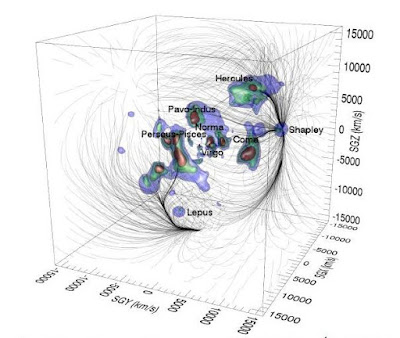Our cosmic neighbourhood,within a cube 200Mpc on each side. The Milky Way is located at the origin of coordinates. There are three isodensity colour contours. The velocity flows are depicted as black lines.
Assuming that cosmic structure formation has been seeded by perturbations sampled from a Gaussian random field, and assuming that the expansion of the universe followed the Lambda-Cold Dark Matter ($\Lambda$CDM) model, the combination of the Wiener filter and Constrained Realization was applied to a sample of galaxy recession velocities along the line-of-sight. The density field in our neighbourhood was then inferred from the reconstructed velocity field.

Some of the most striking images produced by the Cosmicflows team are those which depict the streamlines of the peculiar velocity field in our cosmic neighbourhood. These reveal regions in which the streamlines converge, due to concentrations in the density of matter such as the Great Attractor, and regions in which the streamlines diverge from cosmic voids. In particular, the Cosmicflows team have attempted to identify the surface of divergent points that surrounds us. They define the volume within that watershed as our local galaxy Supercluster, which they name 'Laniakea'.

The Cosmicflows programme assumes only linear deviations from homogeneity and isotropy. This assumption preserves the Gaussian nature of the peculiar velocity field and the density perturbation field. But it does have the consequence that the velocity field is irrotational. i.e, it possesess zero vorticity. Hence, the images of cosmic streamlines contain watersheds, but no whirlpools. Whilst the linear theory should prevail on large scale, the non-linearity should dominate on smaller scales. The Cosmicflows team claim that the non-linearity should only dominate on the Mpc length-scale, and that their approach is therefore valid.A slice through the equatorial plane in the supergalactic coordinate system. The boundary of the Laniakea supercluster is depicted as an orange line. The diagram is shaded with density contours, deep blue regions indicating voids, and red regions indicating zones of high density. The velocity streams within the Laniakea basis of attraction are represented in white.